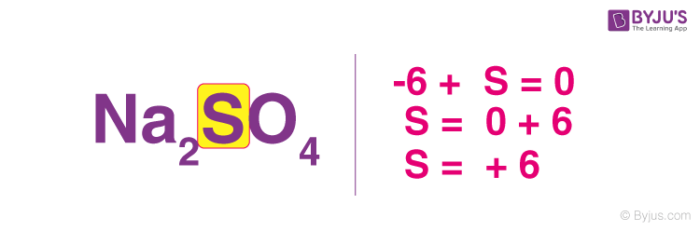Defend yourself better by mastering the science of immunity and vaccines. Secure your free spot, now! Defend yourself better by mastering the science of immunity and vaccines. Secure your free spot, now!

# How To Find Oxidation Number?

In order to learn how to find the oxidation number of an atom in a given compound, it is important to learn what oxidation numbers are. The oxidation number of an atom is a number that represents the total number of electrons lost or gained by it.

## Calculating Oxidation Numbers

An oxidation number can be assigned to a given element or compound by following the following rules.

• Any free element has an oxidation number equal to zero.
• For monoatomic ions, the oxidation number always has the same value as the net charge corresponding to the ion.
• The hydrogen atom (H) exhibits an oxidation state of +1. However, when bonded with an element with less electronegativity than it, it exhibits an oxidation number of -1.
• Oxygen has an oxidation of -2 in most of its compounds. However, in the case of peroxides, the oxidation number corresponding to oxygen is -1.
• All alkali metals (group 1 elements) have an oxidation state of +1 in their compounds.
• All alkaline earth metals (group 2 elements) exhibit an oxidation state of +2 in their compounds.
• In the compounds made up of two elements, a halogen (group 17 elements) have an oxidation number of -1 assigned to them.
• In the case of neutral compounds, the sum of all the oxidation numbers of the constituent atoms totals zero.
• When polyatomic ions are considered, the sum of all the oxidation numbers of the atoms that constitute them equals the net charge of the polyatomic ion.

Thus, the oxidation number of an atom in a given compound can be calculated with the steps mentioned above.

## Solved Examples

In order to help students understand how to find oxidation number, the oxidation states of each individual atom in some example compounds are determined below.An illustration explaining how to find the oxidation number of the sulphur atom in a sodium sulfate molecule can be found above.

### Hydrochloric Acid (HCl)

• As per the rules discussed above, the oxidation state of a group 17 element (halogen) in a diatomic molecule is -1. It is also discussed that hydrogen always exhibits an oxidation number of +1 unless it is paired with a less electronegative element.
• Since chlorine is more electronegative than hydrogen, an oxidation number of +1 can be assigned to the hydrogen atom in HCl.
• Therefore, the oxidation number of hydrogen is +1 and the oxidation of chlorine is -1 in HCl. These values can be verified by adding these oxidation numbers. Since the total is zero, which is the value of the oxidation number corresponding to a neutral molecule, the values are verified.

### Carbon Dioxide (CO2)

• According to the rules to calculate oxidation number, which can be found in the previous subsection, the oxidation number of oxygen in its compounds (excluding peroxides) is -2.
• Since there are two oxygen atoms in carbon dioxide, the total of the oxidation numbers corresponding to each oxygen is -4.
• Since the CO2 molecule is neutral, the carbon atom must exhibit an oxidation state of +4 (the sum of all the oxidation numbers in a neutral molecule is zero).
• Therefore, the oxidation state of oxygen was found to be -2 and the oxidation number of carbon is +4 in a carbon dioxide molecule.

Students can understand how to find oxidation number with the help of the solved examples provided above. To learn more about oxidation states and other related concepts such as oxidizing agents, register with BYJU’S and download the mobile application on your smartphone.

## Frequently Asked Questions – FAQs

Q1

### What is the difference between oxidation state and oxidation number?

The oxidation number differs from the oxidation state in that the oxidation state describes the degree of oxidation of an atom in a molecule, whereas the oxidation number describes the charge that the core metal atom will maintain after all ligands have been removed.

Q2

### Does the oxidation number stay the same?

Most of the oxidation numbers remain the same, and the only oxidation numbers that change are for the compounds that are being oxidised or reduced.

Q3

### Why do we use oxidation numbers?

Oxidation numbers explain an element’s (or compound’s) ability to lose or gain electrons during a chemical reaction.

Q4

### Can oxidation numbers be fractional?

Individual oxidation numbers of elements in a compound (as determined by its structure) can never be fractions. However, an element’s average oxidation number in a compound can be a fraction.

Q5

### What is the oxidation number for a pure element?

The oxidation state of an atom in any pure element, regardless of whether monatomic, diatomic, or polyatomic, is zero. A monatomic ion’s oxidation state is the same as its charge—for example, Na+ = +1, Cl = −1. Fluorine’s oxidation state in chemical compounds is always −1.

Test your knowledge on How To Calculate Oxidation Number